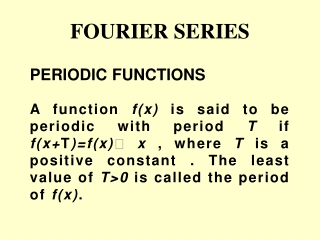DownloadDownload PresentationFOURIER SERIES

# FOURIER SERIES

Télécharger la présentation## FOURIER SERIES

- - - - - - - - - - - - - - - - - - - - - - - - - - - E N D - - - - - - - - - - - - - - - - - - - - - - - - - - -
##### Presentation Transcript

1. FOURIER SERIES A function f(x) is said to be periodic with period T if f(x+T)=f(x) x , where T is a positive constant . The least value of T>0 is called the period of f(x). PERIODICFUNCTIONS

2. f(x+2T) =f ((x+T)+T) =f (x+T)=f(x) f(x+nT)=f(x) for all x Ex.1 f(x)=sin x has periods 2, 4, 6, …. and 2 is the period of f(x). Ex.2 The period of sin nxand cosnxis 2/n.

3. FOURIER SERIES Let be defined in the interval and outside the interval by i.e assume that has the period .The Fourierseries corresponding to is given by

4. where the Fourier coeffecients are

5. If is defined in the interval (c,c+2 ), the coefficients can be determined equivalently from

6. DIRICHLET CONDITIONS Suppose that • f(x) is defined and single valued except possibly at finite number of points in (-l,+l) • f(x) is periodic outside (-l,+l) with period 2l • f(x) and f’(x) are piecewise continuous in(-l,+l)

7. Then the Fourier series of f(x) converges to • f(x) if x is a point of continuity • b)[f(x+0)+f(x-0)]/2 if x is a point of discontinuity

8. METHOD OF OBTAINING FOURIER SERIES OF 1. 2. 3. 4.

9. SOLVED PROBLEMS 1. Expand f(x)=x2,0<x<2 in Fourier series if the period is 2 . Prove that

10. SOLUTION Period = 2 = 2  thus =  and choosing c=0

11. At x=0 the above Fourier series reduces to X=0 is the point of discontinuity

12. 2. Find the Fourier series expansion for the following periodic function of period 4. Solution

13. EVEN AND ODD FUNCTIONS A function f(x) is called odd if f(-x)=-f(x) Ex: x3, sin x, tan x,x5+2x+3 A function f(x) is called even if f(-x)=f(x) Ex: x4, cos x,ex+e-x,2x6+x2+2

14. EXPANSIONS OF EVEN AND ODD PERIODIC FUNCTIONS If is a periodic function defined in the interval , it can be represented by the Fourier series Case1. If is an even function

15. If a periodic function is even in , its Fourier series expansion contains only cosine terms

16. Case 2. When is an odd function

17. If a periodic function is odd in ,its Fourier expansion contains only sine terms

18. SOLVED PROBLEMS 1.For a function defined by obtain a Fourier series. Deduce that Solution is an even function

19. SOLUTION

20. At x=0 the above series reduces to x=0 is a point of continuity, by Dirichlet condition the Fourier series converges to f(0) and f(0)=0

21. PROBLEM 2 Is the function even or odd. Find the Fourier series of f(x)

22. SOLUTION is odd function

23. HALF RANGE SERIES COSINE SERIES A function defined in can be expanded as a Fourier series of period containing only cosine terms by extending suitably in . (As an even function)

24. SINE SERIES A function defined in can be expanded as a Fourier series of period containing only sine terms by extending suitably in [As an odd function]

25. SOLVED PROBLEMS Obtain the Fourier expansion of (x sinx )as a cosine series in .Hence find the value of SOLUTION Given function represents an even function in

26. if

27. in

28. the above series reduces to At is a point of continuity The given series converges to

29. 2) Expand in half range (a) sine Series (b) Cosine series. SOLUTION (a) Extend the definition of given function to that of an odd function of period 4 i.e

30. Here

31. (b) Extend the definition of given function to that of an even function of period 4

32. Exercise problems 1. Find Fourier series of in 2. Find Fourier series of

33. 3.Find the Fourier series of in 4.Find the Fourier series of (-2 ,2) in

34. 5.Represent function In (0,L) by a Fourier cosine series 6.Determine the half range sine series for